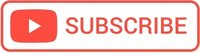Rate this post

# JavaScript Functions

A JavaScript function is defined by keyword function, followed by name, followed by parentheses () and the code to be executed, which is placed inside curly brackets: {}.

function name() {
code to be executed
}


## The function name

The function name should describe what the function will do.

• In Javascript the function name follows the same rules as variables, therefore it can contain letters, numbers, underscores or Dollar sign(\$);
• It must be a single word, can not contain spaces and can not start with a number;

## The function parameters

The function parameters are found between () and can be parameter names separated by commas, as:

function name(parameter1, parameter2) {
code to be executed
}

• The function parameters means data that the function expects to receive it.
You can add as many parameters You want, just separate them with commas;
• Its not necessary to use keyword var inside the parameter list;
• They may be missing also, in this case we have empty parentheses;

## The function invocation

After the function is declared, to execute the code, we have to call(invoke) the function, as:

myFunctionName(); // The function is called

Important!

It does not matter where you call the function in the JavaScript code, because the JavaScript engine reads all code before any function execution.

However, the best practice is to define your functions before you call them!

#### Example

function sayHello(){
}
sayHello();


## The function return

In JavaScript, functions can optionally return values.

For this, we must use the keyword return followed by what we want to return, which can be a variable, a number, or a string.

Be careful!

When Javascript reaches the return statement, the function will stop executing.

## Local Variables

Variables declared within a JavaScript function, are local to the function and can only be accessed from within the function.

    function sayHi(){
var name="John";

}
console.log(name);
// can NOT use name here


## What happens if we pass more parameters to our function?

In other programming languages, this would break your code; in JavaScript, it will just ignore it.

## What happens if we send fewer parameters to our function?

In other programming languages (such as PHP) this would break the code, but the JavaScript engine will just ignore it.

Anyway, is better to declare all variables at the beginning of every scope.

## Default values for function arguments

Is a good practice to provide default values for the arguments that we don’t wanna have to pass in. Otherwise, these arguments will be undefined when our function is called.

In order to define default values for arguments all we have to do is add an equals sign after the argument and then the default value that we want for that argument.

function getPerimeter(length = 15, width = 10) {
let perimeter;
perimeter = 2*(length + width);

return perimeter;
}

getPerimeter(); // 50


Careful! Only the value undefined will trigger the default argument.

# JavaScript function examples

## Calculate the perimeter of a rectangle

function getPerimeter(length, width) {
let perimeter;
perimeter = 2*(length + width);

return perimeter;
}


## Calculate the area of a rectangle

function getArea(length, width) {
let area;
area = length * width;

return area;
}


## Recursive factorial function

A function named factorial that has one parameter: an integer, n and return the value of n! (i.e., factorial).

function factorial(n){
if (n > 1){
return n * factorial(n-1);
}else
return 1;
}


## Write functions using Arrow syntax

Prior to ES6, the main way to define functions was by using the function keyword.

ES6 came along with a more simplistic way of defining functions, namely: the arrow function syntax.